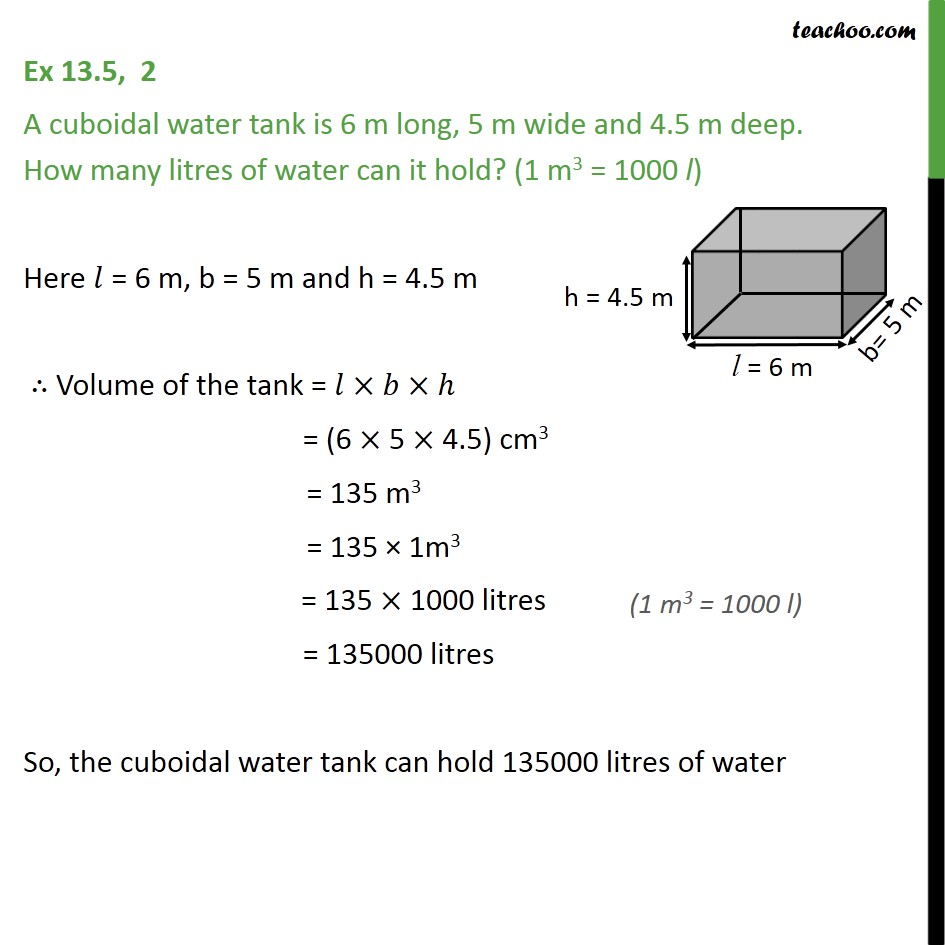1. Chapter 13 Class 9 Surface Areas and Volumes (Term 2)
2. Serial order wise
3. Ex 13.5

Transcript

Ex 13.5, 2 A cuboidal water tank is 6 m long, 5 m wide and 4.5 m deep. How many litres of water can it hold? (1 m3 = 1000 l) Here 𝑙 = 6 m, b = 5 m and h = 4.5 m ∴ Volume of the tank = 𝑙×𝑏×ℎ = (6 × 5 × 4.5) cm3 = 135 m3 = 135 × 1m3 = 135 × 1000 litres = 135000 litres So, the cuboidal water tank can hold 135000 litres of water

Ex 13.5

Chapter 13 Class 9 Surface Areas and Volumes (Term 2)
Serial order wise